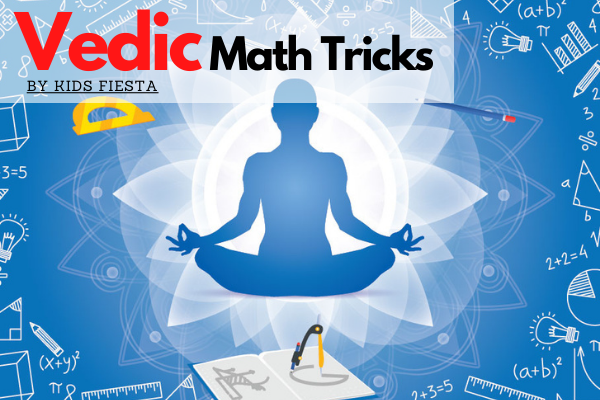# Vedic Math Tricks

Learn the world's fastest Mental Math System

starstarstarstarstar 5.0 (1 ratings)

69 learners enrolled

Language: English

Instructors: Siddharth Khare

Why this course?

## Description

Learn to solve problems through the world's fastest Mental Math System!

Vedic Mathematics is the name given to the ancient system of Indian Mathematics which was rediscovered from the Vedas between 1911 and 1918 by Sri Bharati Krsna Tirthaji (1884-1960).

Perhaps the most striking feature of the Vedic system is its coherence. Instead of a hotch-potch of unrelated techniques the whole system is beautifully interrelated and unified.

The simplicity of Vedic Mathematics means that calculations can be carried out mentally (though the methods can also be written down). There are many advantages in using a flexible, mental system

This course teaches you the fundamentals systems of vedic maths that you can us to solve complex mathematical problems.

When you complete the course training you will be able to solve mathematical calculations in seconds just by mental calculations.

So dive into this course and have fun learning!

# Course Curriculum

 Introduction to the Course Find squares of numbers ending with 5 in seconds. (3:00) Multiplying any number whose units digits add up to 10 in seconds through Vedic Math. (3:00) Multiplying Series of 9 numbers in seconds with Vedic Math. (5:00) Multiplying with a series of 1s Multiply with 11 (5:00) Multiplying with 111 (5:00) Multiply with 1111 (3:00) Multiply any two numbers in seconds through Vedic Maths. (10:00) Criss Cross Method of Multiplication Multiplying two digit numbers Multiplying three digit numbers (5:00) Multiply two 4-digit numbers Multiplying two numbers with unequal digits (4:00) Criss Cross Practice Questions and Answers (2 pages) Division Base Method of Division- Nikhilam Method Dividing 4 Digit Numbers with Nikhilam Method (3:00) Nikhilam Method- Examples Parvartya Method of Division- When the divisor is greater than the base. (6:00) Parvartya Method Tricky Examples (4:00)

# How to Use

After successful purchase, this item would be added to your courses.You can access your courses in the following ways :

• From the computer, you can access your courses after successful login
• For other devices, you can access your library using this web app through browser of your device.

# Reviews

5
star star star star star
people 1 total
 5 1 4 0 3 0 2 0 1 0Launch your Graphy
100K+ creators trust Graphy to teach online